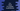# C++ program to check if a number is palindrome or not# Palindrome number check in C++ :

A number is called a palindrome number if its reverse is equal to the number itself. For example, 121 is a palindrome number because it’s reverse 121 is equal to the number.

In this tutorial, we will learn how to check if a number is a palindrome or not in C++. The program will take the number as input from the user, find out if it is palindrome or not and print out one message to the user.

The only way to check if a number is palindrome or not is to reverse the number first and to compare it with the original number. With this program, you will learn how to read user inputs and how to use loops in C++.

## C++ program :

``````#include <iostream>
using namespace std;

int getReverseNumber(int no)
{
// 3
int rev = 0;
int rightmostDigit;

// 4
while (no != 0)
{
rightmostDigit = no % 10;
rev = (rev * 10) + rightmostDigit;
no /= 10;
}
return rev;
}

int main()
{
// 1
int n;
cout << "Enter a number : " << endl; cin >> n;

// 2
if (n == getReverseNumber(n))
{
cout << n << " is a palindrome number" << endl;
}
else
{
cout << n << " is not a palindrome number" << endl;
}
return 0;
}``````

### Explanation :

The commented numbers in the above program denote the step numbers below :

1. Create one integer variable n. Ask the user to enter a number. Read it and store it in n.
2. Find out the reverse of this number using function getReverseNumber and check if both are equal. If equal, print that this number is a palindrome number, else it is not a palindrome number.
3. getReverseNumber takes one integer as its argument and it returns the reverse value for that integer. To find the reverse, we are picking the rightmost digit of the number one by one and adding it to a different variable. rev is to store the reverse value and rightmostDigit is to hold the right most digit of the current number.
4. In this step, we are using one while loop. This loop will run until the value of no becomes 0. Inside the loop, we are finding out the right most digit of the number and assigning this value to rightmostDigit. Next, we are adding this value to the end of rev and finally, we are removing it from no. This method returns the reverse number i.e. rev.

### Sample Output :

``````Enter a number :
1234321
1234321 is a palindrome number

Enter a number :
1123456
1123456 is not a palindrome number

Enter a number :
12345654321
2147483647 is not a palindrome number``````

## Method 2: By converting the number to a string :

We can also check for palindrome by converting one number to a string and comparing it with its reversed value like below :

``````#include <iostream>
#include <cstring>
using namespace std;

int main()
{
int n;
cout << "Enter a number : " << endl; cin >> n;

string str = to_string(n);
bool isPalindrome = true;

int j = strlen(str.c_str()) - 1;
for (int i = 0; i < j; i++, j--)
{
if (str[i] != str[j])
{
isPalindrome = false;
break;
}
}

if (isPalindrome)
{
cout << n << " is a palindrome number" << endl;
}
else
{
cout << n << " is not a palindrome number" << endl;
}
return 0;
}``````

Here, the strlen method is used to convert the number to a string. Using the for loop, we are checking if each character is equal to the character that is exactly opposite to it if we divide the string in half.

The output of this program is the same as the above program.